Subscribe to our newsletter to receive the latest news and events from TWI:

# Numerical Analysis of Contact Stresses and Fatigue Damage

## Numerical Analysis of Contact Stresses Between Mooring Chain Links and Potential Consequences for Fatigue Damage

Philippe Bastid and Simon D Smith
TWI Ltd, Cambridge, UK

Paper Presented at OMAE 2013 Paper No 11360

## Abstract

Elastic-plastic finite element analyses of the proof loading have been performed. These analyses have shown that the proof loading also generates very high tensile residual stresses in the region surrounding the interlink contact zone. This region also experiences significant in-service cyclic stresses under cyclic tension or out-of-plane bending. The combination of the cyclic stresses and high tensile residual stress is of concern and it is proposed that the periphery of the interlink contact zone should be carefully reviewed. It is understood that chain link fatigue at present is only based on the risk of fatigue damage at the KT point.

This paper presents and discusses results of finite element stress analyses of studless chains of different sizes and grades, and show the relative fatigue sensitivity of the KT and contact regions. The chain grade, dimensions and loading regime are shown to be important.

## 1. Introduction

For many years, mooring chains have been designed per well established standards such as API RP 2SK (1), Lloyds Register (2) or DNV-OS-E301 (3). These standards help engineers to select the relevant chain link grade and size depending on the fatigue loads and the planned life of the mooring system. These codes are mainly based on mechanical testing and experience (1-3). It is understood that their recommendations aim to avoid fatigue failure, mainly at two different locations: the crown and the KT point; the KT point is approximately the region of the intrados where the shank and the crown intersect (Figure 1). These regions are those where the ratio between the local stress and the nominal stress in the shank is the highest. The stress ratio is determined from an elastic analysis of the chain link under tension. For example, the stress ratio at KT is about 4 for a standard chain link.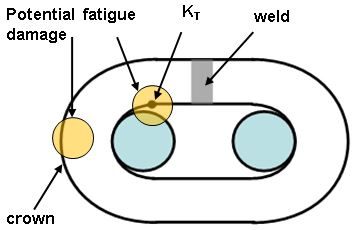Figure 1 Regions of the chain link where high stress ranges can cause fatigue cracking

Mooring failures are a major concern when subsequent investigations show that the chain’s design and manufacture followed the recommended standards. The occurrence of recent failures prompted a number of projects whose objectives were to understand the failure mechanisms and to produce data for establishing further design criteria. One Joint Industrial Project (JIP) on out-of-plane bending (OPB) of mooring chain links was led by Single Buoy Moorings (SBM). This project highlighted the need for analyses of OPB, which had not been previously addressed by the standards.

Standards also seem to ignore the possibility of fatigue damage arising from stress cycles in the region of the interlink contact zone when the loading is purely tensile. The work described below gives results from a study based on finite elements models, where the local stress cycles are combined with predicted residual stresses due to proof loading. The objective was to determine if a purely tensile fatigue load could cause cracking in the interlink contact zone like the one shown in Figure 2.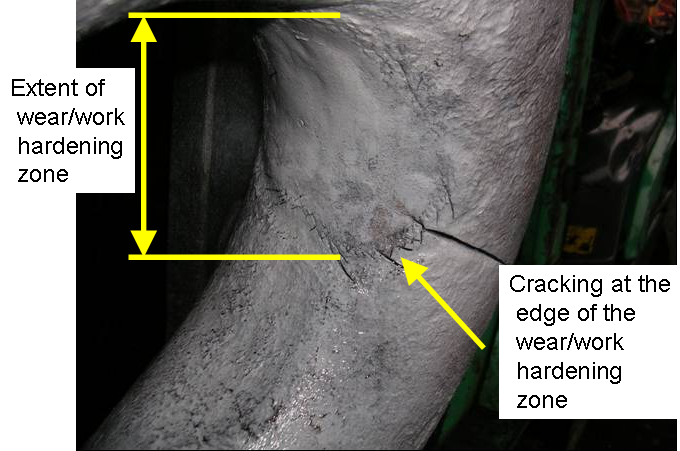Figure 2 Cracks observed at the periphery of the interlink contact zone.

Models were prepared and analyses performed using the finite element analyses (FEA) package Abaqus v. 6.12.3.

## 2. Nomenclature

 D wire diameter L link length W link breadth σy material yield stress σUTS material tensile strength σeng engineering stress σtrue true stress εeng engineering strain εtrue true strain

## 3. Finite Element Models

### 3.1 Geometry

Only one quarter of the two links were analysed, and symmetry conditions were applied at the nodes lying on symmetry planes (Figure 3).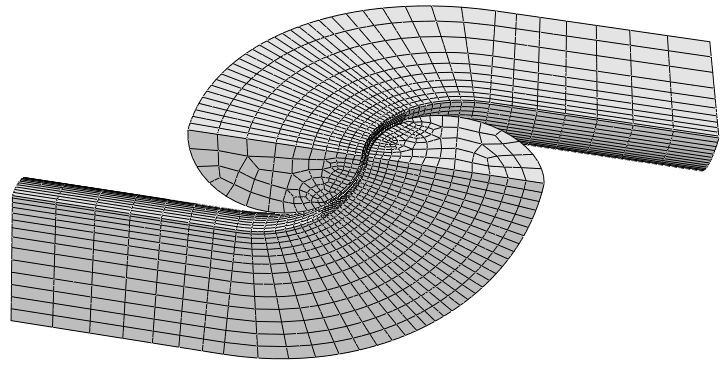Figure 3 Geometry and mesh of the models analysed. The figure shows the 124mm (D) size chain links.

An idealized geometry of each link was assumed. They were therefore modelled using cylindrical and toroidal volumes having circular cross sections. The variation of the cross section of the wire due to bending during manufacture was therefore not taken into account in these analyses.

Three studless link geometries were investigated, corresponding to nominal wire diameters D equal to 76, 124 and 162mm. The links were assumed to be of standard dimension, where the length L and breadth W are given by:

L=6D ,                    W=3.35D                                [Eq. 1]

The models were meshed with quadratic 3D solid elements with reduced integration. In order to refine the mesh, in the region of the contact zone, each link was partitioned into two instances which were perfectly tied together (Figure 4).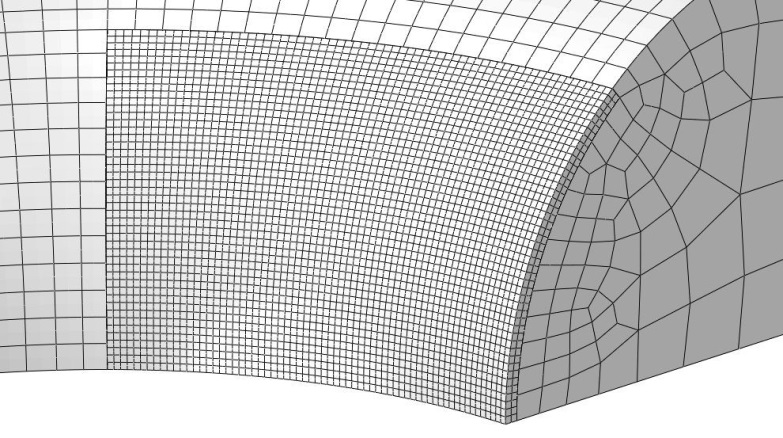Figure 4 Views of the mesh of one chain link showing the refined mesh in the contact zone.

### 3.2 Materials’ Behaviour

Three materials were investigated, corresponding to Grades R3S, R4, and R5. R3S and R4 were chosen because they are commonly used in existing mooring systems. R5 was analysed in order to determine any benefit in using this relatively new grade.

The materials were assumed to be elastic-plastic, with linear isotropic work hardening. The yield stress values of the materials were not chosen to be equal to the minimum specifications (1-3), but to be more representative of real values issued from tensile tests. In the plastic behaviour assumed, the flow stress increases linearly from the yield stress to the UTS of the material, and remains constant when the plastic strain is above the minimum elongation specified for each grade.

Because of the large deformation due to compression in the contact zone, the stress – strain data were converted into true stress – true strain data using the equations:

εtrue = ln(1+εeng)          σtrue = σeng (1+εeng)       [Eq. 2]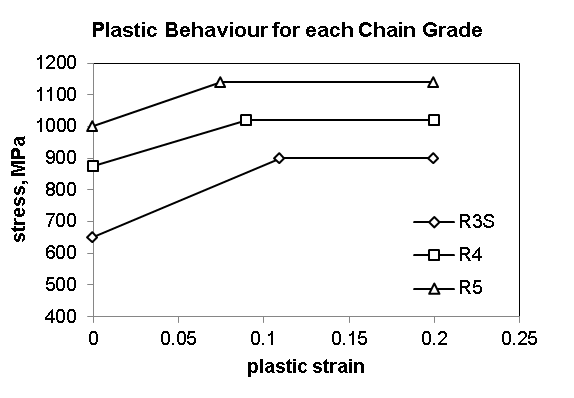Figure 5 Material's behaviour used in the analyses

Similarly, the “non-linear geometry” option of the FEA software was used so that the geometry of the link was updated during each calculation increment. The true stress – true strain curves and the parameters for the plastic behaviour are shown in Figure 5.

Three symmetry planes were defined in accordance to the geometry and loading of the model. The displacement of the nodes lying on the faces highlighted in the figure is constrained in the direction normal to the planes (Figure 6).

The load was applied to a reference point that was coupled the face at the centre of the second link (Figure 6). The nodes lying on this face are constrained so that their displacement in the direction of the chain axis is uniform across the face. This constraint defines the model’s periodicity.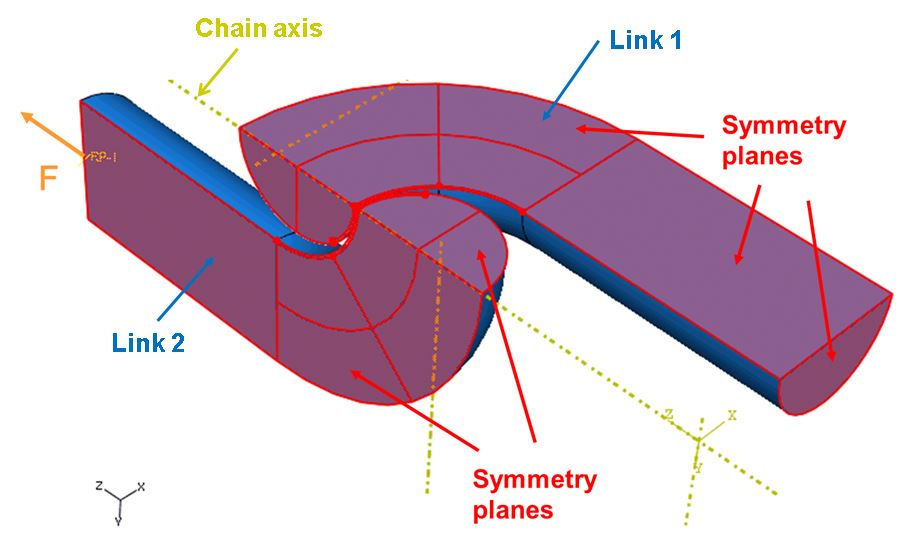Figure 6 Symmetry planes and reference point where the tensile load was applied in the model.

The load histories were determined following the specifications for the chain size and grade. For each of the five cases analysed, the loading history was as summarized below:

• 20% MBL (maximum cyclic load)
• 0% MBL (residual stress)

The residual stress field, obtained when the load is completely released after proof loading, was calculated in order to determine if local tensile values can be high enough to cause damage before service. It is worth noting that the model of the chain link was permanently under tensile loading during service. The minimum load of the fatigue cycle, mainly caused by the dead weight of the chain, was assumed to be 10% of MBL rather than zero.

The resulting loads for each case are summarised in Table 1.

Table 1 Tensile loads applied to the 5 chain models analysed

kN
76 R4 4201 1200 600
124 R3S 9134 2610 1305
124 R4 10051 2872 1436
124 R5 11738 3354 1677
162 R4 15624 4464 2232

Frictional contact was assumed between the links, with a contact coefficient of 0.4.

## 4. Results

### 4.1 Preamble

The results obtained for each of the models are qualitatively similar. Therefore, the figures mainly show the contour plots obtained from the analysis of the 124mm R4 grade links, and quantitative results are given for each case in tables.

Figure 7 shows the Von Mises stress contour plot when the link is at the proof load. The extent of yielding (where the Von Mises stress is above 875MPa) is significant. Only a small band of the shanks is still in the elastic domain. This figure shows that yielding under tension occurs in the region of the KT point and in the crown.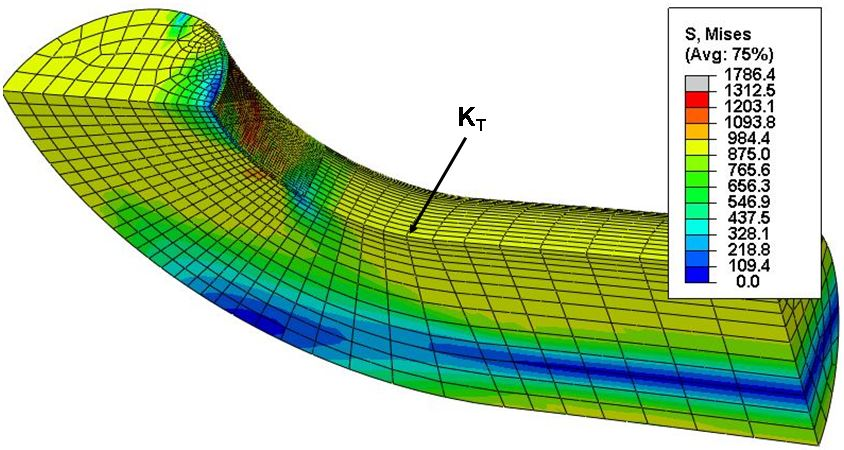Figure 7 Von Mises stress contour plot when the proof load is applied. The yield stress used for R4 grade is 875MPa

The residual stress distribution after complete unloading is shown in Figures 8 to 10 and reported in Table 2. Five regions of particular interest are defined on these figures:

• Region 1 is the KT point;
• Region 2 is at the crown on the link’s axis;
• Region 4 is the intrados at the edge of the zone of contact during proof loading;
• Region 5 is near the intrados, out of the contact zone.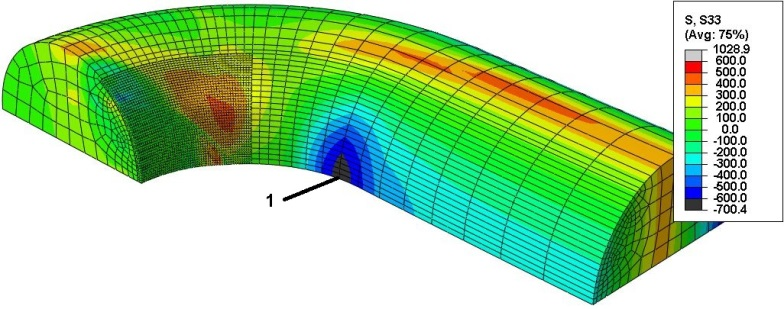Figure 8 Axial stress after unloading from the proof load, showing region 1 (KT), where the residual stress is compressive (124mm R4).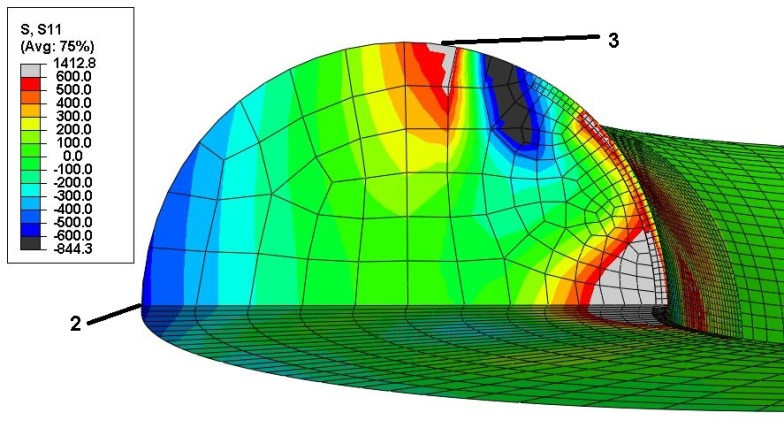Figure 9 Hoop stresses at the crown after unloading from the proof load, showing regions 2 and 3, where the residual stress is compressive at 2 and tensile at 3 (124mm R4).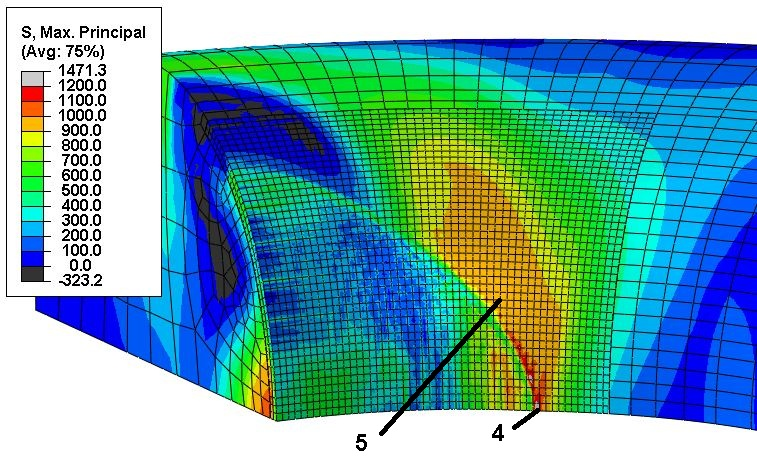Figure 10 Maximum principal stress in the contact zone after unloading from the proof load showing regions 4 and 5, where the residual stress is tensile (124mm R4)

Table 2 Residual stress after release of proof load obtained from the cases analysed.

mm
MPa
Regions12345
76 R4 -805 -478 695 1236 843
124 R3S -650 -325 715 991 590
124 R4 -674 -526 633 1207 852
124 R5 -794 -590 710 1512 942
162 R4 -563 -510 622 1200 864

### 4.3 Fatigue Stress Range

The minimum load of the fatigue cycle was assumed to be 10% of MBL (static load). The maximum load was assumed to be 20% of MBL. The stress contour plots for each load case are shown in Figure 11 to 14, and the stress values after proof loadingare reported in Table 3.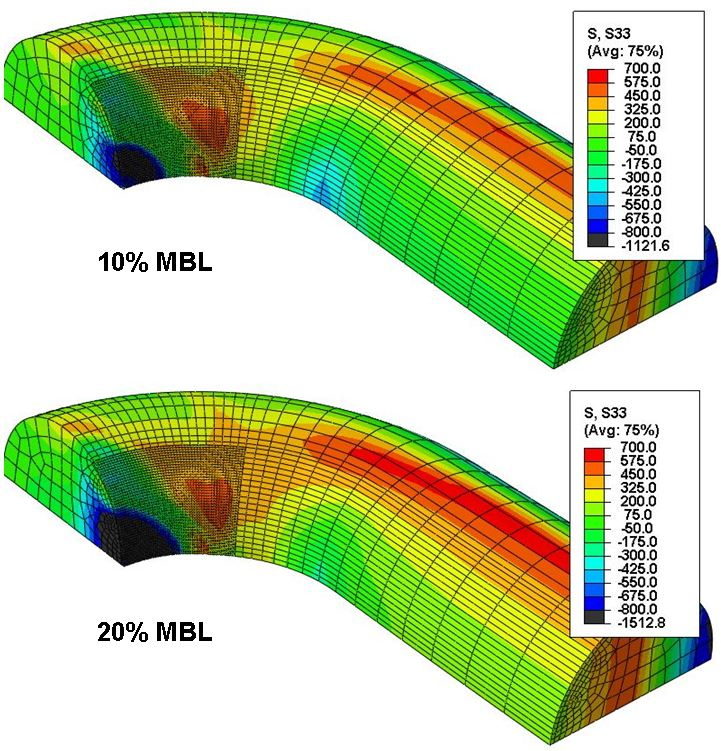Figure 11 Variation of the axial stress in Region 1 between the minimum load (10% MBL - upper) and the maximum load (20% MBL - lower) of the fatigue cycle (124mm R4).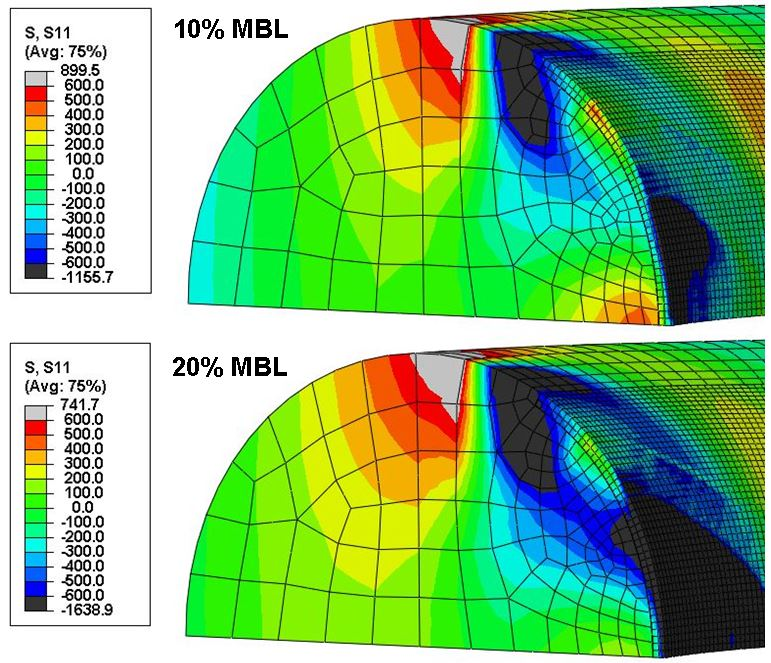Figure 12 Stresses in direction X in Regions 2 and 3 at the minimum load (10% MBL - upper) and the maximum load (20% MBL - lower) of the fatigue cycle (124mm R4).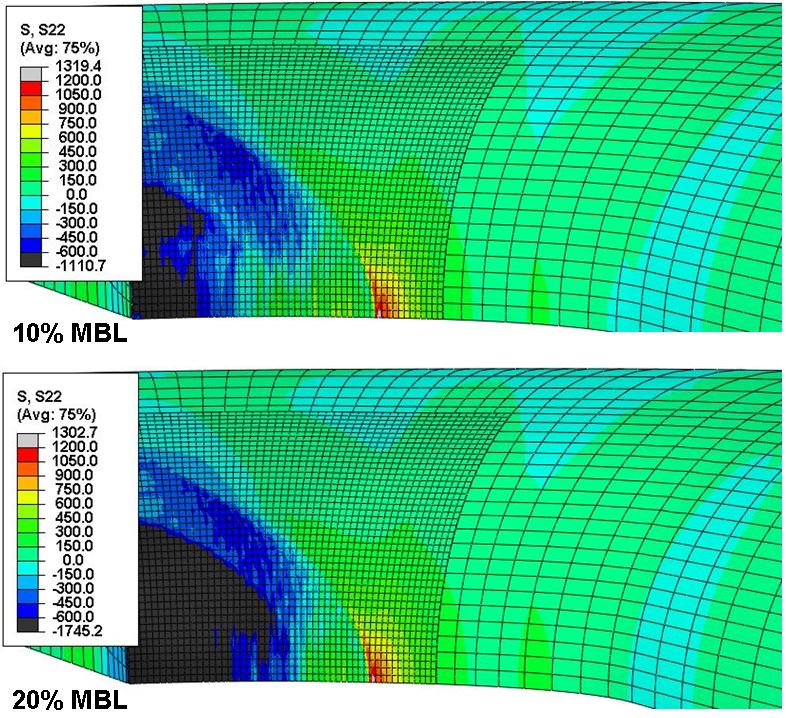Figure 13 Stresses in direction Y in Region 4 at the minimum load (10% MBL - upper) and the maximum load (20% MBL - lower) of the fatigue cycle (124mm R4).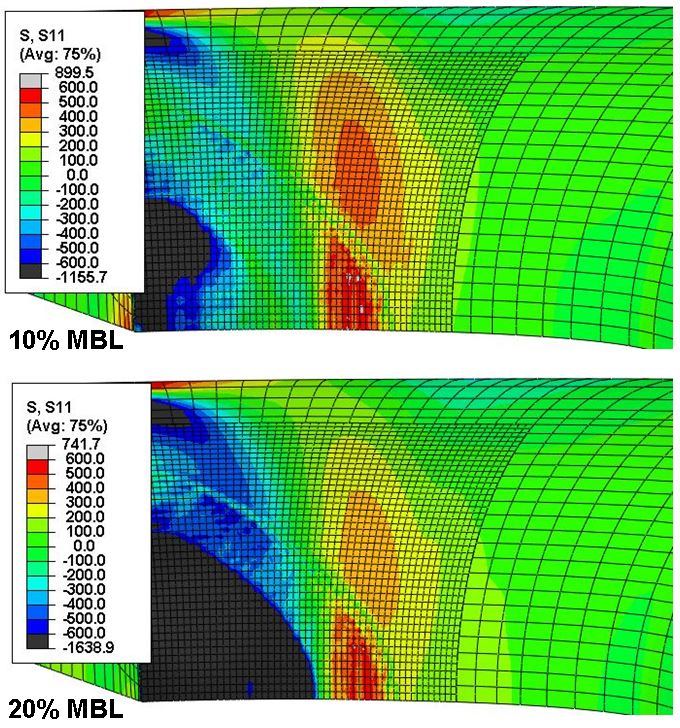Figure 14 Stresses in direction X in Region 5 at the minimum load (10% MBL - upper) and the maximum load (20% MBL - lower) of the fatigue cycle (124mm R4).

The orientation of the stress shown in Figures 11 to 13 is identical or very close to the direction of maximum principal stress for the regions labelled. For these 4 regions, the direction of maximum principal stress for both load cases was similar to the maximum principal direction of the difference between both stress fields. However, in the case of Region 5 (Figure 14), the direction was chosen to facilitate the post-processing, and does not correspond to the maximum principal stress, which will be higher than the stress in direction X reported in Table 3.

Table 3 Stress at the 5 regions of interest for 10% MBL and 20% MBL, and corresponding stress range.

CaseRegionStress at 10% MBL MPaStress at 20% MBL MPaStress range
MPa
Tensile part
MPa
D76
R4
1 -525 -261 264 0
2 -202 36 238 36
3 -8 187 195 187
4 1242 1243 1 1
5 102 7 95 95
D124 R3S 1 -428 -221 207 0
2 -116 62 178 62
3 -13 139 152 139
4 1005 1000 5 5
5 52 4 48 48
D124 R4 1 -424 -186 228 0
2 -268 -38 230 0
3 -5 175 180 175
4 1218 1203 15 15
5 147 7 140 140
D124 R5 1 -502 -225 276 0
2 -291 -26 265 0
3 -2 207 209 207
4 1509 1493 14 14
5 149 3 146 146
D162 R4 1 -334 -110 224 0
2 -271 -45 226 0
3 18 190 172 172
4 1195 1189 6 6
5 191 6 185 185

## 5. Discussion

### 5.1 Residual Stress

The results in Table 2 confirm the benefit of proof loading for regions 1 and 2. In these regions, the residual stress is predicted to be compressive, which will improve the fatigue life. However, in regions 3, 4, and 5, at the periphery of the contact zone, the residual stress is tensile and can reach very high values. These residual stress data need to be analysed together with the local stress cycle due to the subsequent cyclic loading.

The residual stress in region 4 is high. However, because the local stress cycle shown in Table 3 appears to be small (about 15MPa at the highest), fatigue damage is unlikely.

The tensile residual stress in region 3 is below the yield stress of the material. However this stress is tensile, so any defect would be open during the whole of any loading cycle. Therefore the whole local stress range would cause fatigue crack propagation. Region 5 is similar to region 3. However, it is worth noting that increasing the load from 10% of MBL to 20% of MBL results in increasing the stress in region 3, but decreases the stress in region 5.

### 5.2 Fatigue Stress Range

The stress ranges reported in Table 3 are, in general, directly affected by the loads applied in each case. The fatigue life prediction of mooring chains presented by API RP 2SK (1) uses a load range divided by the value of the minimum breaking load of ORQ chain links of the same size. Therefore, the size of the link can be accounted for in the fatigue design. This assumes that the definition of the MBL varies in accordance with the improvement of the fatigue behaviour from one grade to another.

In order to facilitate the analysis of the stress range data, similar scaling has been applied, where the scaling factor is the nominal stress in the shank at MBL. For the cases 76mm R4, 124mm R3S, 124mm R4, 124mm R5 and 162mm R4, the nominal stress values are 661MPa, 540MPa, 594MPa, 694MPa, and 541MPa respectively. Table 4 gives the stress range from Table 3 scaled by the nominal stress. In region 5, the operation was applied to the maximum principal value of the difference between the stress tensors, since the maximum stress value was higher than the stress range in direction 1 reported in Table 3.

The scaled stress ranges obtained for locations 1 to 4 do not vary significantly between different cases. This shows that the fatigue life predicted from FEA results for each of these locations would not be significantly dependent on the size and grade of the chain. This is only true if the grade and size are chosen in agreement with the service loads expected (ie 10% - 20% of MBL load range).

However, a significant increase of the scaled stress range with size is predicted in region 5. In the case of the 162mm size link, the scaled stress range becomes very close to the value at the KT point. So it would seem that the two locations should both be considered when chain design standards are prepared. An increase is also predicted when the grade is higher, but to a lesser extent. The higher stress range in this region is likely to cause the occurrence of fatigue cracking at the periphery of the contact zone, such as that shown in Figure 2. Because the scale stress range increases with size, the design curve from API is not fully conservative. Chains designed per this standard are safe if the links are of small or medium dimension, but the possibility of extra recommendations needs to be considered for the larger links.

Table 4 Stress range at the 5 regions of interest scaled by the nominal stress in the shank at MBL.

CaseRegionStress range
MPa
Scaled stress range
D76 R4 1 264 0.40
2 238 0.36
3 195 0.30
4 1 0.00
5 117 0.18
D124 R3S 1 207 0.38
2 178 0.33
3 152 0.28
4 5 0.01
5 63 0.12
D124 R4 1 228 0.38
2 230 0.39
3 180 0.30
4 15 0.03
5 156 0.26
D124 R5 1 276 0.40
2 265 0.38
3 209 0.30
4 14 0.02
5 178 0.30
D162 R4 1 224 0.41
2 226 0.42
3 172 0.32
4 6 0.01
5 205 0.38

The highest stress range is predicted in region 1. However, if the sign of the local stress at minimum and maximum service load is taken into account, the predicted life of region 5 may be shorter than that of region 1. It is suggested, for fatigue assessment, that an effective stress range can be calculated by accounting for 100% of the tensile part of the stress range, and 60% of the compressive part of the stress range (6). If this recommendation is applied to the predicted stress values, the location of the highest damage would be region 3 for smaller links, but would move to region 5 when the size of the link increases. The regions at risk of damage would also be dependent on the load range, or on the minimum service load. If both these quantities increase, the stress cycle in regions 1 and 2 would be less compressive, whereas the stress cycles in region 5 would be less tensile, so that the risk of cracking in regions 1 and 2 becomes higher than in region 5. Region 3 is always tensile, and the probability of fatigue cracking at this location, compared to other locations, remains the same irrespective of mean load.

Two main assumptions have been made when defining these models. In the analyses presented here, the work hardening was assumed to increase linearly, until a “cut-off” value corresponding to the UTS of the materials. Also, the work hardening was modeled as being isotropic. Using kinematic hardening would lead to different results when non-monotic loads are applied to the model. Ideally, low cycle fatigue stress-strain curves should be used to determine the proportion of kinematic and isotropic hardening in the plastic behaviour.

The chain links were assumed to be stress free before proof loading. However, a number of manufacturing stages have been applied to the initial wire: bending, heat treatment, quenching, and eventually shot peening. All these have an effect on the residual stress. A better prediction of the maximum and minimum stress values could be obtained if all these stages were taken into account in the analyses.

This work addressed the case of mooring chain links under pure tensile loading. If out-of-plane bending or twisting takes place, the local stress cycles are different to those investigated here, but the residual stress distribution remains the same.

Finally, these results have not been validated experimentally. Results from finite elements models may be used in chain designs, or in a future update of standards. Experimental measurements of residual stresses, or service stresses, must be carried out on links whose manufacturing was well controlled in order to give confidence in the modelling results, or to improve the models.

## 6. Conclusions

Five models of chain links have been prepared, covering a range of grades and sizes. The loads applied to these models were the proof load, followed by two tensile services loads, equal to 10% of MBL and 20 % of MBL. The distribution of residual stress, and the stress range caused by the 10% MBL load cycles have been analysed at 5 locations that were identified to be at risk of cracking. The main conclusions of these analyses are:

• Proof loading generates compressive and tensile residual stresses. High tensile residual stresses at the periphery of the contact zone can be detrimental to fatigue life.
• The cyclic stress range in Region 5 near the contact zone was found to increase quite significantly with the size of the link. Therefore the design curve from API may be unconservative when applied to large chain links.
• In large chains, fatigue cracking is likely to first occur at the periphery of the contact zone (Region 5) when the service loads are between 10% of MBL and 20% of MBL. If the service load or maximum fatigue load are higher, local stress cycles may overcome compressive residual stresses, and cracking may first occur at other locations, such as the KT point or the crown.
• For smaller chain links, the fatigue stress range in Region 5 is less damaging than in the other regions investigated, and cracking would occur preferentially in the crown or at KT, whatever the load range and mean load.
• These observations are based on finite element analyses results only. More precise conclusions based on the exact location of crack initiation, depending on chain grade, size, load range and mean load, require further validation work and modelling using accurate data relevant to the size and grade of chain to be investigated.

## 7. Acknowledgments

TWI would like to thank BP for funding the studies that initiated a series of projects on finite element analyses of contact stresses in mooring chain links, whose main results are summarized in this paper.

## 8. References

• API RP 2SK: ‘Design, analysis and maintenance of moorings for floating production systems’, 1996.
• Lloyds Register: ‘Rules and regulations for the classification of ships: Manufacture, testing and certification of materials’, 1999.
• Offshore Standards DNV-OS-E301 – Position mooring, 2004.
• Tipton S M, Shoup G J: ‘The effect of proof loading on the fatigue behaviour of open link chain’, J. of Eng. Mat. and Tech., Trans. of ASME, Vol. 114, pp 27-33, 1992
• Calas Lopes Pacheco P M, Kenedi P P, Ferreira Jorge J C, ‘Elastoplastic analysis of the residual stress in chain links’, Proceedings of OMAE 2002, Paper OMAE2002-28083.
• BS7608: ‘Code of practice for fatigue design and assessment of steel structures’, 1993.